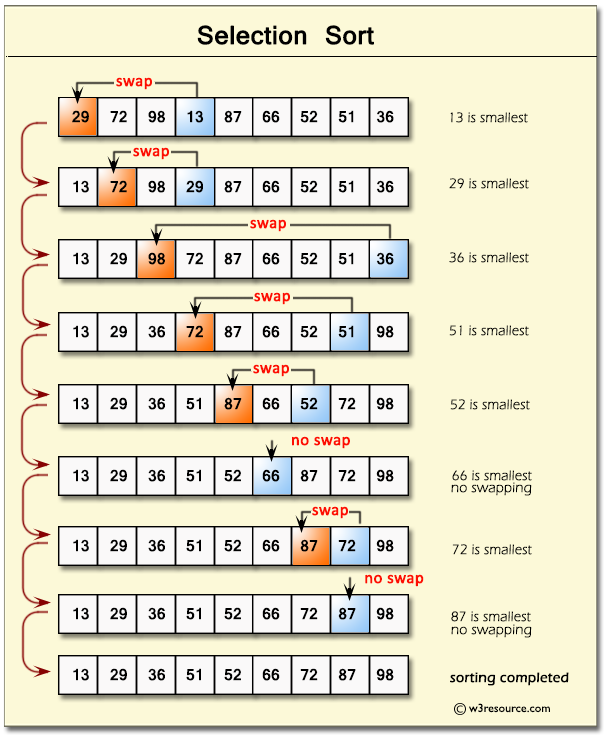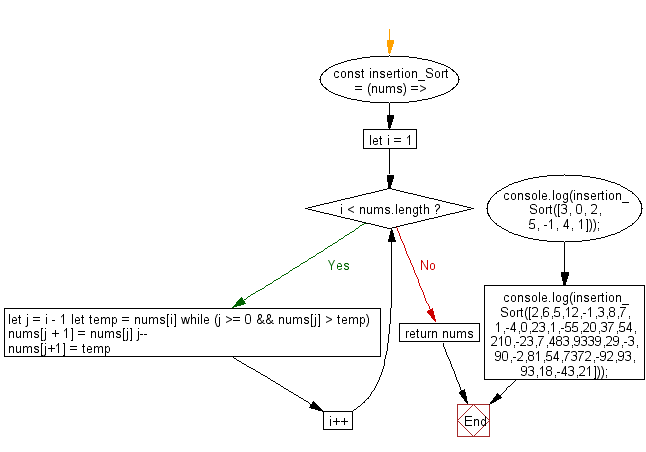﻿ PHP: Sort a list of elements using Selection sort - w3resource

# PHP Searching and Sorting Algorithm: Selection sort

## PHP Searching and Sorting Algorithm: Exercise-4 with Solution

Write a PHP program to sort a list of elements using Selection sort.

The selection sort improves on the bubble sort by making only one exchange for every pass through the list.

Pictorial Presentation : Selection SortSample Solution :

PHP Code :

``````<?php

function selection_sort(\$data)
{
for(\$i=0; \$i<count(\$data)-1; \$i++) {
\$min = \$i;
for(\$j=\$i+1; \$j<count(\$data); \$j++) {
if (\$data[\$j]<\$data[\$min]) {
\$min = \$j;
}
}
\$data = swap_positions(\$data, \$i, \$min);
}
return \$data;
}

function swap_positions(\$data1, \$left, \$right) {
\$backup_old_data_right_value = \$data1[\$right];
\$data1[\$right] = \$data1[\$left];
\$data1[\$left] = \$backup_old_data_right_value;
return \$data1;
}
\$my_array = array(3, 0, 2, 5, -1, 4, 1);
echo "Original Array :\n";
echo implode(', ',\$my_array );
echo "\nSorted Array :\n";
echo implode(', ',selection_sort(\$my_array)). PHP_EOL;
?>
```
```

Sample Output:

```Original Array :
3, 0, 2, 5, -1, 4, 1
Sorted Array :
-1, 0, 1, 2, 3, 4, 5
```

Flowchart :PHP Code Editor:

Have another way to solve this solution? Contribute your code (and comments) through Disqus.

What is the difficulty level of this exercise?

Test your Programming skills with w3resource's quiz.

﻿